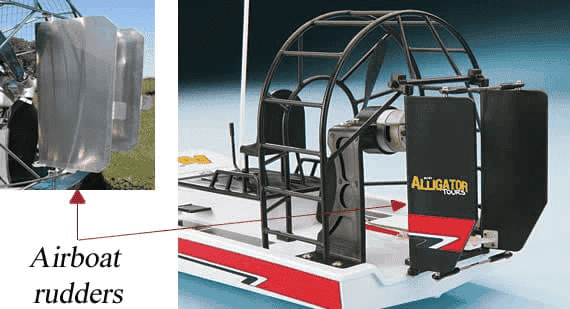# Grade 11 Question: Newton's Third Law -- Calculating acceleration of an airboat

Ibo7526
Homework Statement:
c) How can the fan on the airboat be used to change the direction the boat is travelling in?
How can it be used to increase or decrease the speed of the boat?
d) If the boat is going upstream against a current that has a force of 250 N against the
motion of the boat, find the acceleration of the boat if there is a forward force of 360 N from
the fan. (Remember that the mass of the boat is 220 kg.)
Relevant Equations:
Fnet=F1+ F2
Fnet=ma
a=Fnet/m
for c) i need help
d)
Given: Let [forward] be positive.
F 1 = — 250 N
F 2 = 360 N
Unknown: a = ?
Equation: F net= F1+ F2
F net= m a
Solve: F net= (— 250 N) + 360 N = 110 N
F net= m a
a = F net/ m
= 110 N / 220 kg= 0.5 m/s [forward]
Statement: The acceleration of the boat is 0.5 m/s [forward].

Last edited by a moderator:

Mentor
Welcome to PhysicsForums.Homework Statement:: c) How can the fan on the airboat be used to change the direction the boat is traveling in?
How can it be used to increase or decrease the speed of the boat?

for c) i need help

Google Images is often a good resource for questions like this. I did a Google Images search on airboat steerting, and quickly got to this helpful website:

https://www.flatbottomboatworld.com/how-do-airboats-work/•PeroK
•